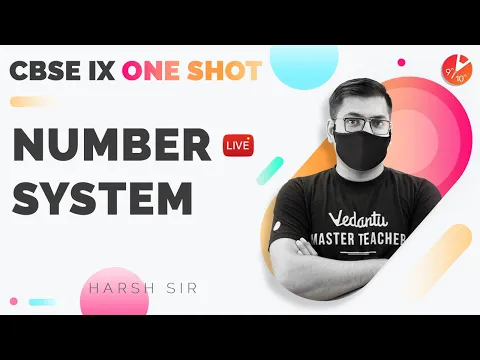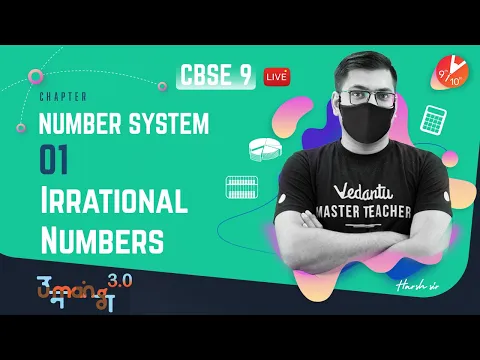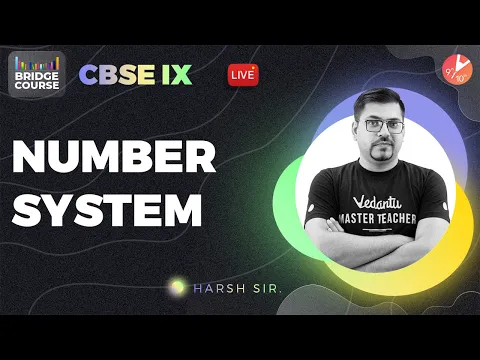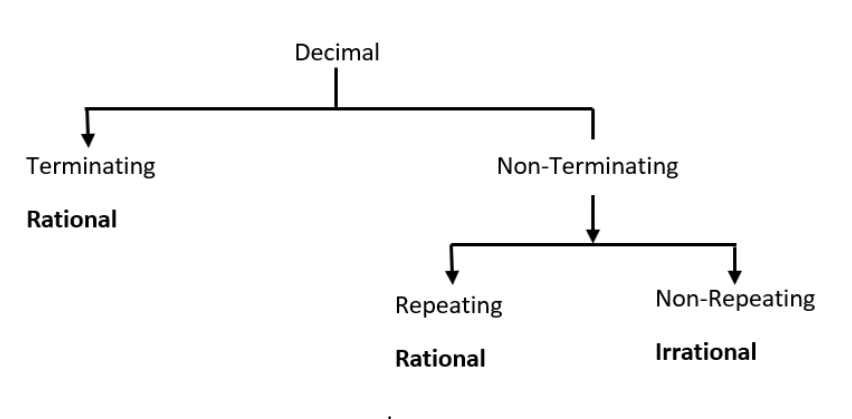Courses
Courses for Kids
Free study material
Free LIVE classes
More

# NCERT Solutions for Class 9 Maths Chapter 1 - ExerciseLIVE
Join Vedantu’s FREE Mastercalss

## CBSE Class 9 NCERT Solutions Maths Chapter 1 Exercise 1.3

### Important Points to Note before Solving Exercise 1.3:

Decimal Expansions of Rational and Irrational Numbers

A. For rational numbers, the decimal expansion is either terminating or non-terminating and recurring.

For example: 1/2 = 0.5 , 1/3 = 3.33…….

B. For an irrational number, the decimal expansion is non-terminating and non-recurring.

Example: √2 = 1.41421356..

### Expressing Decimals as Rational Numbers

• The term terminating decimal expansion refers to the fact that the decimal representation or expansion ends after a given number of digits. The decimal expansion of 1/16, for example, ends after four digits.

• The non-terminating but recurring decimal expansion means that, although having an infinite number of digits, the decimal representation has a recurrent pattern. In 1/13, for example, the quotient is 0.0769230769..., which is a recurring decimal quotient.

• Even if the repetition begins after a given number of digits, any non-terminating yet recurring decimal representation corresponds to a rational number.

Last updated date: 29th Sep 2023
Total views: 514.5k
Views today: 5.14k
Watch videos on
NCERT Solutions for Class 9 Maths Chapter 1 - ExerciseNumber System in One Shot | CBSE Class 9 Maths Chapter 1 | CBSE lX - One Shot | Vedantu 9 and 10
Vedantu 9&10
SubscribeShare
11.5K likes
257K Views
2 years ago
Play QuizNumber System L-1 | Irrational Numbers | CBSE Class 9 Maths Chapter 1 | Umang 2021 | Vedantu 9 & 10
Vedantu 9&10
9K likes
186.6K Views
2 years agoNumber System | CBSE Class 9 Maths Chapter 1 - Bridge Course 🎯 | Harsh Sir | Vedantu 9 and 10
Vedantu 9&10
7.2K likes
143.8K Views
2 years ago

## Access NCERT Solutions for Class 9 Maths Chapter 1 – Number System

### Exercise (1.3)

1. Write the following in decimal form and say what kind of decimal expansion each has:

(i) $\dfrac{ {36}}{ {100}}$

Ans: Divide $36$ by $100$.

$\begin{matrix} &{0.36}\\ 100&{\overline{)\;36\quad}}\\ &\underline{-0\quad}\\ &360\\ &\underline{-300\quad}\\ &\;\;600\\ &\underline{-600}\\ &\underline{\quad 0 \;\;} \end{matrix}$

So, $\dfrac{36}{100}=0.36$ and it is a terminating decimal number.

(ii) $\dfrac{ {1}}{ {11}}$

Ans: Divide $1$ by $11$.

$\begin{matrix} &{0.0909..}\\ 11&{\overline{)\;1\quad}}\\ &\underline{-0\quad}\\ &10\\ &\underline{\;\;-0\quad}\\ &\;\;100\\ &\underline{\;-99}\\ &\quad10\\ &\quad\underline{\;\;-0\quad}\\ &\;\;100\\ &\underline{\;-99}\\ &\quad1\quad\\ \end{matrix}$

It is noticed that while dividing $1$ by $11$, in the quotient $09$ is repeated.

So, $\dfrac{1}{11}=0.0909.....$ or

$\dfrac{1}{11}=0.\overline{09}$

and it is a non-terminating and recurring decimal number.

(iii)  ${4}\dfrac{ {1}}{ {8}}$

Ans: $4\dfrac{1}{8}=4+\dfrac{1}{8}=\dfrac{32+1}{8}=\dfrac{33}{8}$

Divide $33$ by $8$.

$\begin{matrix} &{4.125}\\ 8&{\overline{)\;33\quad}}\\ &\underline{-32\quad}\\ &10\\ &\underline{\;\;-8\quad}\\ &\;\;20\\ &\underline{-16}\\ &\;\quad\;40\\ &\quad\underline{\quad-40\quad}\\ &\quad\underline{\quad 0\quad}\\ \end{matrix}$

Notice that, after dividing $33$ by $8$, the remainder is found as $0$.

So, $4\dfrac{1}{8}=4.125$ and it is a terminating decimal number.

(iv)  $\dfrac{ {3}}{ {13}}$

Ans: Divide $3$ by $13$.

$\begin{matrix} &{0.230769}\\ 13&{\overline{)\;3\quad}}\\ &\underline{-0\quad}\\ &30\\ &\underline{\;-26\quad}\\ &\;40\\ &\underline{-39\quad}\\ &\;\quad\;10\\ &\quad\underline{\quad-0\quad}\\ &\quad{\quad100}\\ &\quad\quad\underline{-91\quad}\\ &\quad\quad90\\ &\quad\quad\underline{-78\quad}\\ &\quad\quad 120\\ &\quad \quad\underline{-117\quad}\\ &\quad\quad\underline{\quad 3\quad} \end{matrix}$

It is observed that while dividing $3$ by $13$, the remainder is found as $3$ and that is repeated after each $6$ continuous divisions.

So, $\dfrac{3}{13}=0.230769.......$ or

$\dfrac{3}{13}=0.\overline{230769}$

and it is a non-terminating and recurring decimal number.

(v)   $\dfrac{ {2}}{ {11}}$

Ans: Divide $2$ by $11$.

$\begin{matrix} &{0.1818}\\ 11&{\overline{)\;2\quad}}\\ &\underline{-0\quad}\\ &20\\ &\underline{\;-11\quad}\\ &\;90\\ &\underline{-88\;}\\ &\;\quad\;20\\ &\quad\underline{\quad-11\quad}\\ &\quad{\quad90}\\ &\quad\underline{-88}\\ &\quad2\\ \end{matrix}$

It can be noticed that while dividing $2$ by $11$, the remainder is obtained as $2$ and then $9$, and these two numbers are repeated infinitely as remainders.

So, $\dfrac{2}{11}=0.1818.....$ or

$\dfrac{2}{11}=0.\overline{18}$

and it is a non-terminating and recurring decimal number.

(vi) $\dfrac{ {329}}{ {400}}$

Ans: Divide $329$ by $400$.

$\begin{matrix} &{0.8225}\\ 400&{\overline{)\;329\quad}}\\ &\underline{-0\quad}\\ &3290\\ &\underline{\;-3200\quad}\\ &\;900\\ &\underline{-800\;}\\ &\quad\;1000\\ &\quad\underline{\quad-800\quad}\\ &\quad{\quad2000}\\ &\quad\underline{\quad-2000\quad}\\ &\quad\underline{\quad 0 \quad}\\ \end{matrix}$

It can be seen that while dividing $329$ by $400$, the remainder is obtained as $0$.

So, $\dfrac{329}{400}=0.8225$ and is a terminating decimal number.

2. You know that $\dfrac{ {1}}{ {7}} {=0} {.142857}...$. Can you predict what the decimal expansions of $\dfrac{ {2}}{ {7}} {,}\dfrac{ {3}}{ {7}} {,}\dfrac{ {4}}{ {7}} {,}\dfrac{ {5}}{ {7}} {,}\dfrac{ {6}}{ {7}}$  are, without actually doing the long division? If so, how?

(Hint: Study the remainders while finding the value of $\frac{ {1}}{ {7}}$ carefully.)

Ans: Note that,  $\dfrac{2}{7},\dfrac{3}{7},\dfrac{4}{7},\dfrac{5}{7}$ and $\dfrac{6}{7}$ can be rewritten as $2\times \dfrac{1}{7},\text{ 3}\times \dfrac{1}{7},\text{ 4}\times \dfrac{1}{7},\text{ 5}\times \dfrac{1}{7},$ and $6\times \dfrac{1}{7}$

Substituting the value of $\dfrac{1}{7}=0.142857$ , gives

$2\times \dfrac{1}{7}=2\times 0.142857...$$=0.285714...$

$3\times \dfrac{1}{7}=3\times 0.428571...$$\text{=}\,\text{0}\text{.428571}...$

$4\times \dfrac{1}{7}=4\times 0.142857...$$\text{=}\,\text{0}\text{.571428}...$

$5\times \dfrac{1}{7}=5\times 0.71425...$  $\text{=}\,\text{0}\text{.714285}...$

$6\times \dfrac{1}{7}=6\times 0.142857...$$\text{=}\,\text{0}\text{.857142}...$

So, the values of $\dfrac{2}{7},\text{ }\dfrac{3}{7},\text{ }\dfrac{4}{7},\text{ }\dfrac{5}{7}$ and $\dfrac{6}{7}$ obtained without performing long division are

$\dfrac{2}{7}=0.\overline{285714}$

$\dfrac{3}{7}=0.\overline{428571}$

$\dfrac{4}{7}=0.\overline{571428}$

$\dfrac{5}{7}=0.\overline{714285}$

$\dfrac{6}{7}=0.\overline{857142}$

3. Express the following in the form $\dfrac{ {p}}{ {q}}$, where ${p}$ and ${q}$ are integers and ${q}\ne {0}$.

(i) ${0} {.}\overline{ {6}}$

Ans: Let $x=0.\overline{6}$

$\Rightarrow x=0.6666$                                                   ….… (1)

Multiplying both sides of the equation (1) by $10$, gives

$10x=0.6666\times 10$

$10x=6.6666$…..                 …… (2)

Subtracting the equation $\left( 1 \right)$ from $\left( 2 \right)$, gives

\begin{align} & 10x=6.6666..... \\ & \underline{-x=0.6666.....} \\ & 9x=6 \\ & 9x=6 \\ & x=\dfrac{6}{9}=\dfrac{2}{3} \\ \end{align}

So, the decimal number becomes

$0.\overline{6}=\dfrac{2}{3}$  and it is in the required  $\dfrac{p}{q}$ form.

(ii) ${0} {.}\overline{ {47}}$

Ans: Let  $x=0.\overline{47}$

$\text{ }\Rightarrow x=0.47777.....$                                             ……(a)

Multiplying both sides of the equation (a) by $10$, gives

$10x=4.7777.....$         ……(b)

Subtracting the equation $\left( a \right)$ from $\left( b \right)$, gives

\begin{align} & 10x=4.7777..... \\ & \underline{-x=0.4777.....} \\ & 9x=4.3 \\ \end{align}

Therefore,

\begin{align} & \,\,\,\,\,\,x=\dfrac{4.3}{9}\times \dfrac{10}{10} \\ & \Rightarrow x=\dfrac{43}{90} \\ \end{align}

So, the decimal number becomes

$0.\overline{47}=\dfrac{43}{90}$  and it is in the required $\dfrac{p}{q}$ form.

(iii) ${0} {.}\overline{ {001}}$

Ans: Let $x=0.\overline{001}\Rightarrow$           …… (1)

Since the number of recurring decimal number is $3$, so multiplying both sides of the equation (1) by $1000$, gives

$1000\times x=1000\times 0.001001.....$ …… (2)

Subtracting the equation (1) from (2) gives

\begin{align} & 1000x=1.001001..... \\ & \underline{\text{ }-x=0.001001.....} \\ & 999x=1 \\ \end{align}

$\Rightarrow x=\dfrac{1}{999}$

Hence, the decimal number becomes

$0.\overline{001}=\dfrac{1}{999}$ and it is in the $\dfrac{p}{q}$ form.

4. Express ${0} {.99999}.....$ in the form of $\dfrac{ {p}}{ {q}}$ . Are you surprised by your answer? With your teacher and classmates, discuss why the answer makes sense.

Ans:

Let $x=0.99999.....$                                                             ....... (a)

Multiplying by $10$ both sides of the equation (a), gives

$10x=9.9999.....$                                                             …… (b)

Now, subtracting the equation (a) from (b), gives

\begin{align} & 10x=9.99999..... \\ & \underline{\,-x=0.99999.....} \\ & \,\,9x=9 \\ \end{align}

$\Rightarrow x=\dfrac{9}{9}$

$\Rightarrow x=1$.

So, the decimal number becomes

$0.99999...=\dfrac{1}{1}$ which is in the $\dfrac{p}{q}$ form.

Yes, for a moment we are amazed by our answer, but when we observe that $0.9999.........$ is extending infinitely, then the answer makes sense.

Therefore, there is no difference between $1$ and $0.9999.........$ and hence these two numbers are equal.

5. What can the maximum number of digits be in the repeating block of digits in the decimal expansion of $\dfrac{ {1}}{ {17}}$ ? Perform the division to check your answer.

Ans: Here the number of digits in the recurring block of $\dfrac{1}{17}$ is to be determined. So, let us calculate the long division to obtain the recurring block of $\dfrac{1}{17}$. Dividing $1$ by $17$ gives

Thus, it is noticed that while dividing $1$ by $17$, we found $16$ number of digits in the

repeating block of decimal expansion that will continue to be $1$ after going through $16$ continuous divisions.

Hence, it is concluded that $\dfrac{1}{17}=0.0588235294117647.....$ or

$\dfrac{1}{17}=0.\overline{0588235294117647}$ and it is a recurring and non-terminating decimal number.

6. Look at several examples of rational numbers in the form $\dfrac{ {p}}{ {q}}\left( {q}\ne {0} \right)$, where ${p}$ and ${q}$ are integers with no common factors other than ${1}$ and having terminating decimal representations (expansions). Can you guess what property ${q}$ must satisfy?

Ans: Let us consider the examples of such rational numbers $\dfrac{5}{2},\dfrac{5}{4},\dfrac{2}{5},\dfrac{2}{10},\dfrac{5}{16}$ of the form $\dfrac{p}{q}$ which have terminating decimal representations.

\begin{align} & \dfrac{5}{2}=2.5 \\ & \dfrac{5}{4}=1.25 \\ & \dfrac{2}{5}=0.4 \\ & \dfrac{2}{10}=0.2 \\ & \dfrac{5}{16}=0.3125 \\ \end{align}

In each of the above examples, it can be noticed that the denominators of the rational numbers have powers of $2,5$ or both.

So, $q$ must satisfy the form either ${{2}^{m}}$, or ${{5}^{n}}$, or  both ${{2}^{m}}\times {{5}^{n}}$ (where $m=0,1,2,3.....$ and $n=0,1,2,3.....$) in the form of $\dfrac{p}{q}$.

7. Write three numbers whose decimal expansions are non-terminating non-recurring.

Ans: All the irrational numbers are non-terminating and non-recurring, because irrational numbers do not have any representations of the form of $\dfrac{p}{q}$ $\left( q\ne 0 \right)$, where $p$ and $q$are integers. For example:

$\sqrt{2}=1.41421.....$,

$\sqrt{3}=1.73205...$

$\sqrt{7}=2.645751....$

are the numbers whose decimal representations are non-terminating and non-recurring.

8. Find any three irrational numbers between the rational numbers $\dfrac{ {5}}{ {7}}$ and $\dfrac{ {9}}{ {11}}$.

Ans: Converting  $\dfrac{5}{7}$and $\dfrac{9}{11}$ into the decimal form gives

$\dfrac{5}{7}=0.714285.....$ and

$\dfrac{9}{11}=0.818181.....$

Therefore, $3$ irrational numbers that are contained between $0.714285......$ and $0.818181.....$

are:

\begin{align} & 0.73073007300073...... \\ & 0.74074007400074...... \\ & 0.76076007600076...... \\ \end{align}

Hence, three irrational numbers between the rational numbers $\dfrac{5}{7}$ and $\dfrac{9}{11}$ are

\begin{align} & 0.73073007300073...... \\ & 0.74074007400074...... \\ & 0.76076007600076...... \\ \end{align}

9. Classify the following numbers as rational or irrational:

(i) $\sqrt{ {23}}$

Ans: The following diagram reminds us of the distinctions among the types of rational and irrational numbers.After evaluating the square root gives

$\sqrt{23}=4.795831.....$ , which is an irrational number.

(ii) $\sqrt{ {225}}$

Ans: After evaluating the square root gives

$\sqrt{225}=15$, which is a rational number.

That is, $\sqrt{225}$ is a rational number.

(iii) ${0} {.3796}$

Ans: The given number is $0.3796$. It is terminating decimal.

So, $0.3796$ is a rational number.

(iv) ${7} {.478478}$

Ans: The given number is $7.478478\ldots .$

It is a non-terminating and recurring decimal that can be written in the $\dfrac{p}{q}$ form.

Let      $x=7.478478\ldots .$                                   ……(a)

Multiplying the equation (a) both sides by $100$ gives

$\Rightarrow 1000x=7478.478478.....$                                               ……(b)

Subtracting the equation (a) from (b), gives

\begin{align} & 1000x=7478.478478.... \\ & \underline{\text{ }-x=\text{ }7.478478\ldots .} \\ & 999x=7471 \\ & 999x=7471 \\ & \text{ }x=\dfrac{7471}{999} \\ \end{align}

Therefore, $7.478478.....=\dfrac{7471}{999}$, which is in the form of $\dfrac{p}{q}$

So, $7.478478...$ is a rational number.

(v) ${1} {.101001000100001}.....$

Ans: The given number is $1.101001000100001....$

It can be clearly seen that the number $1.101001000100001....$ is a non-terminating and non-recurring decimal and it is known that non-terminating non-recurring decimals cannot be written in the form of $\dfrac{p}{q}$.

Hence, the number $1.101001000100001....$ is an irrational number.

Vedantu is your best choice when it comes to getting all the Exercise 1.3 Class 9 Maths question 3 Solutions and NCERT Solutions for all exercises and chapters. The Solutions will naturally help you a great deal before your examinations. You can use it for brushing up on the concepts while also preparing for the examinations in a smarter way. You can access tips, tricks, step by step preparation guides and shortcut techniques along with the Solutions. These will help you a great deal with regard to understanding more about the problems and Solutions. This will also help you revise on the basic formulas and concepts when you take them up just before the examinations.

Practice makes a person perfect and these NCERT Solutions are a great way to practice Mathematics prior to taking the actual examination. The NCERT Maths Class 9 Chapter 1 Exercise 1.3 are given in a concise way by Vedantu. These Solutions are prepared by experienced and skilled subject matter experts and teachers who have very good experience in the subject. They know the kind of questions that you will have to encounter in the examination. You will naturally be able to prepare better for the examination since you will encounter many types of mathematical problems and questions. NCERT Solutions are always excellent reference points for students. The Maths NCERT Solutions Class 9 Chapter 1 Exercise 1.3 offers an extensive look at real numbers and their decimal expansion. This is vital for your overall understanding of the subject in the future as well. The NCERT Solutions will have step-wise dissemination of all the answers for questions that are present in the exercises. Everything is based upon the CBSE curriculum and NCERT guidelines. This covers the whole syllabus and helps you score more marks in your examination.

### NCERT Solutions for Class 9 Maths Chapter 1 Other Exercises

 Chapter 1 - Number System Other Exercises in PDF Format Exercise 1.1 4 Questions & Solutions Exercise 1.2 2 Questions & Solutions Exercise 1.4 2 Questions & Solutions Exercise 1.5 5 Questions & Solutions Exercise 1.6 3 Questions & Solutions

### Why Vedantu Helps you a Great Deal

It’s not just about Exercise 1.3 in Chapter 1 for Class 9 Maths. At Vedantu, you get access to free PDFs for all subjects and chapters in your syllabus. You can always benefit from the carefully created NCERT Solutions which are designed to help you understand better and, solve problems faster. These Solutions will help you revise and stay on track for your upcoming examination and you can expect to score higher as well. The Solutions are based upon NCERT guidelines which means that there is no confusion on your part.

You will be able to get insights regarding all the important questions which are likely to be faced by you in the examination. This is because our teachers know the types of questions that come in CBSE examinations and they will help you prepare accordingly. You should keep practising these innovatively designed NCERT Solutions for Maths in Class 9 in order to build your understanding of core concepts and also improve your foundation for Class 10. This is where the convenience of Vedantu’s free PDFs will please you immensely. You can download these PDFs anytime and keep practising at home without having to go through the course content again and again.

Maths is one of the most important subjects in Class 9 and with the right guidance and insights, you can score really good marks in the examination. Moreover, with regular practice, the fear of numbers faced by many students can be easily overcome. Vedantu is a reliable study Solution in this regard. You can count on Vedantu for NCERT Solutions, resources, tools and valuable guidelines for all your subjects. Vedantu strives to ensure smart and innovative study (NCERT) Solutions for students which help them learn faster and score higher marks in their examinations.

## FAQs on NCERT Solutions for Class 9 Maths Chapter 1 - Exercise

1. Give an overview of the chapter Number system.

In this Chapter, you will get to understand about the different types of numbers along with their varied characteristics. The chapter primarily deals with irrational numbers, real numbers and their decimal expansion. It is followed by representing real numbers on the numbers, operating on real numbers, and lastly laws of exponents for real numbers.

You will also be able to learn about rational numbers and irrational numbers with their properties. The chapter also introduces you to:

• Classification of expressions into rational or irrational numbers

• Simplification of expressions

• Number Line representation

• Rationalization

2. Give a brief on decimal and decimal classification.

Decimal is a very interesting and fun part of the Number System. Decimal fractions were first introduced and used by Chinese at the end of the 4th century and then spread to the Middle East and from there to Europe. Decimals are used in our daily life without consciousness. For instance – counting of money, filling fuel into our vehicle or while measuring our weight. Decimals can never be whole numbers. Few examples: 1.8, 000.23 etc.

Decimals classifications: Terminating decimal fractions are 17/4= 4.25, 21/5 = 4.2 and so on.

Non - terminating decimal fractions are 16/3 = 5.33333 , 15.35353535 etc.

Integer: All the numbers that does not have decimals in them are known as integers. Thus, -9, 4, 1476 etc. Do you know? All integers are whole numbers including the negative numbers.

3. What does this chapter mainly focuses on?

The chapter mainly focuses on different types of numbers. They are:

• Complex Number

• Imaginary Number

• Real Number

• Rational Number

• Irrational Numbers

• Integers

• Whole Numbers

• Natural Numbers

• Natural Numbers - any of the given numbers that are used for counting purpose, starting from one, is considered a natural number.

• Whole Numbers - the total union set of all the Natural numbers which includes zero are the set of whole numbers.

• Integers - the set of all the whole numbers including their negative terms is called the set of integers.

• Rational Numbers - any number which can be written as a ratio of two natural numbers is known as a rational number.

• Irrational Numbers - any number which cannot be written in the form of a ratio of two natural numbers is known as an irrational number.

4. How will Vedantu’s NCERT solutions of class 9 maths chapter 1 number system will help us?

Solutions to the questions are prepared by our science experts as per the latest guidelines provided by the CBSE and NCERT. We have drafted the solutions in a step by step way to make your preparation simple and easy to understand. We have given all the relevant exams and diagrams to the questions with real-life examples.

These solutions are in a systematic way which helps you in building up a strong base of all the concepts. We make sure that all the topics and sub-topics are covered from every chapter and we also have designed these solutions in a way to make your learning process more fun, fascinating and enjoyable.

5. Are the Vedantu NCERT Solutions for Chapter 1 of Class 9 Maths helpful?

Vedantu is the best website for students to get solutions for their NCERT subjects. These solutions are really helpful for the students because they are very accurate according to the NCERT pattern. Also, they help students prepare for examinations in a better way. Solutions of Chapter 1 of Class 9 Maths by Vedantu offers comprehensive solutions, making learning much easier for students.

6. Where can I download solutions for Chapter 1 of Class 9 Maths?

7. How can I score good marks in Chapter 1 of Class 9 Maths?

You can score good marks in the Chapter 1 of Class 9 Maths in the following ways-

• Do not be in doubt regarding any concept. Concepts are very important when it comes to Maths.

• Learn the formulas of each chapter very thoroughly. Formulae are the key to solving all kinds of Math problems.

• Solve each and every question of the exercises at the end of each chapter. The more you practise the better you can get at Maths.

• Refer to the solutions for a better understanding of a chapter. The solution will contribute to a better understanding of each concept.

8. How many chapters are there in Class 9 Maths apart from Chapter 1?

The NCERT Class 9 Maths has a total of 13 chapters. All these chapters are a combination of the Basic Number Systems, Algebra, Geometry, And Mensuration. All of these 13 chapters are equally important for us to learn. For all of these chapters, you can get free solutions on Vedantu. You can download the chapter-wise solutions from Vedantu in PDF format free of cost.

9. What are the important chapters in Class 9 Maths apart from Chapter 1?

All the 13 chapters in Class 9 Maths are important. Do not leave out a single chapter when you are preparing for your examinations. All the chapters carry a certain amount of weightage in your exams. Also, leaving out even a single chapter will make you struggle to understand other chapters. So studying all the chapters is important. Other than that, you will get questions from all of the chapters as a part of your final exam.